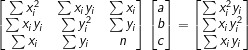# Rational Function Regression Calculator

You can use this rational function regression calculator to determine the rational function equation that is best fitted to a given set of points.

Simply follow the two steps outlined below to use the calculator.

1. Complete the respective fields with the known X and Y variables.
2. Click on the "Calculate" button to generate the rational function regression equation.

If you wish to clear the results to compute the results for a different set of values, click on the "Reset" button.

Rational Function Regression Calculator

## Rational Function Regression: y = (ax + c)/(x − b)

The ratio of the two linear functions represents one of the most straightforward rational functions. Rational functions that take the form y = (ax + c)/(x − b) represent a good method of modeling any data that levels off after a given time period without any oscillations. The horizontal asymptote of a rational function is y = a, while the vertical asymptote is x = b, and the y-intercept is −c/b.

When a function takes the form y = (ax + c)/(x − b), the a, b, and c parameters are not linear. However, it is possible to transform the equation through the use of simple algebra:

y = (ax + c)/(x − b)

(x − b)y = ax + c

xy − by = ax + c

xy = ax + by + c

This equation does not incorporate linear a, b, and c variables; as such, it is not possible to apply the least-squares method to identify the "best fit" values a, b, and c values; i.e., you can minimize the equation.

F(a, b, c) = ∑(xiyi − axi − byi − c)2,

This is tantamount to solving the system as follows:

∂F/∂a = 0, ∂F/∂b = 0, and ∂F/∂c = 0

The solution can be determined using matrix math.

## Using Matrices to Determine a, b, and c

The matrix equation that can be employed for simple rational regression is as follows:where n is the number of data pairs (xi, yi), and ∑ the summation sign.

Providing the three-by-three matrix presented on the left is invertible, a unique solution (a, b, c) can be employed to minimize the function F(a, b, c) and delivers the parameters for the best fit rational function.

Example:

Identify the equation of a rational function that fits the points (x, y):

(4, 3), (2, 4), (3, 6)

Through the use of this rational function regression calculator, you can delineate the following equation:

y = (3.6x − 12)/(x − 3.2)

This equation represents a reasonably good fit for the data.

You may also be interested in our Linear Regression Calculator or Quadratic Regression Calculator